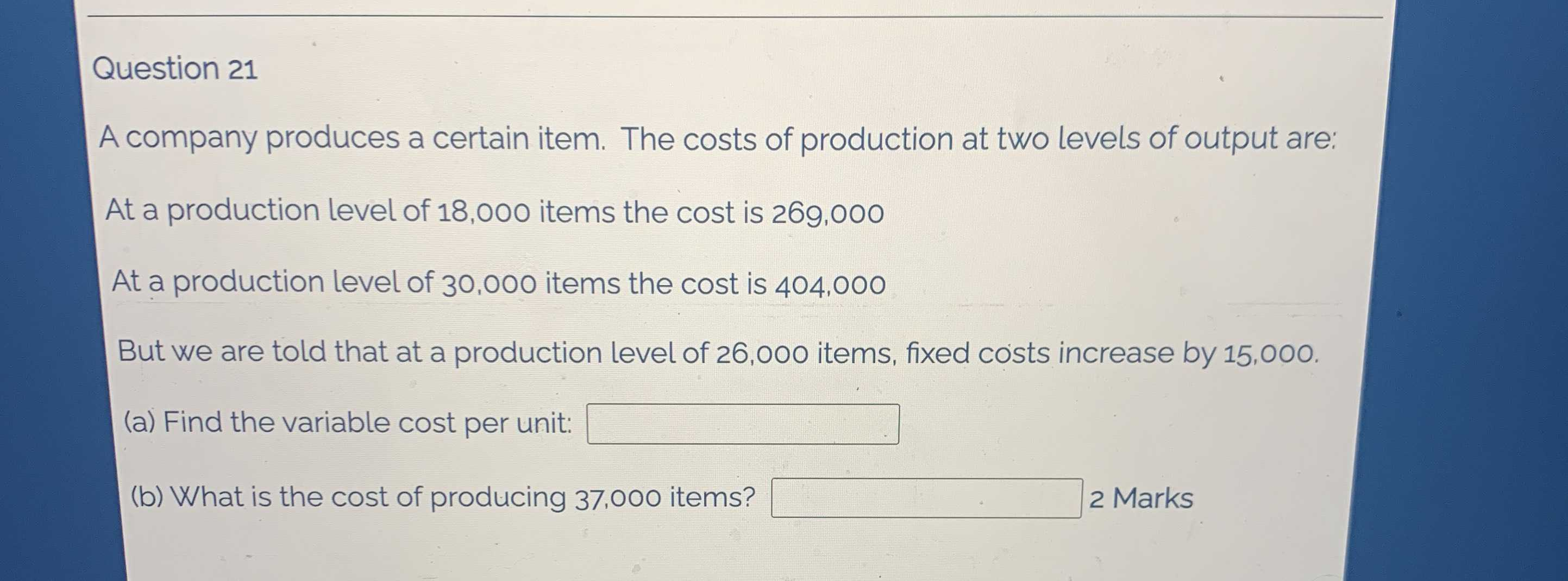### Still have math questions?

Algebra
QuestionA company produces a certain item. The costs of production at two levels of output are: At a production level of $$18,000$$ items the cost is $$269,000$$

At a production level of $$30,000$$ items the cost is $$404,000$$

But we are told that at a production level of $$26,000$$ items, fixed costs increase by $$15,000$$ .

(a) Find the variable cost per unit: (b) What is the cost of producing $$37,000$$ items?